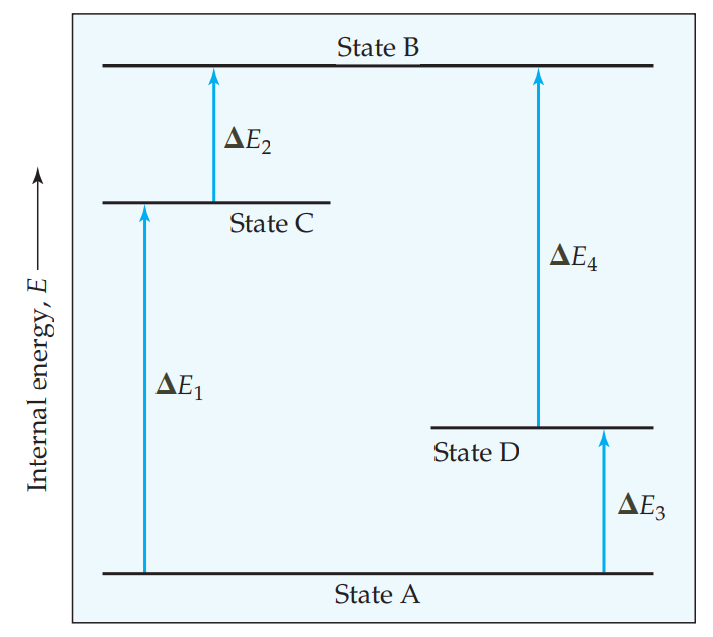×
Get Full Access to Chemistry: The Central Science - 12 Edition - Chapter 5 - Problem 6e
Get Full Access to Chemistry: The Central Science - 12 Edition - Chapter 5 - Problem 6e

×

# Visualizing ConceptsThe diagram shows four states of aISBN: 9780321696724 27

## Solution for problem 6E Chapter 5

Chemistry: The Central Science | 12th Edition

• Textbook Solutions
• 2901 Step-by-step solutions solved by professors and subject experts
• Get 24/7 help from StudySoup virtual teaching assistantsChemistry: The Central Science | 12th Edition

4 5 1 393 Reviews
26
3
Problem 6E

The diagram shows four states of a system, each with different internal energy, E. (a) Which of the states of the system has the greatest internal energy? (b) In terms of theE values, write two expressions for the difference in internal energy between State A and State B. (c) Write an expression for the difference in energy between State C and State D. (d) Suppose there is another state of the system, State E, and that its energy relative to State A is. Where would State E be on the diagram? [Section 5.2]Step-by-Step Solution:
Step 1 of 3

Problem 6EVisualizing ConceptsThe diagram shows four states of a system, each with different internal energy, E.(a) Which of the states of the system has the greatest internal energy(b) In terms of the E values, write two expressions for the difference in internal energybetween State A and State B.(c) Write an expression for the difference in energy between State C and State D.(d) Suppose there is another state of the system, State E, and its energy relative to State A isE = E1 + E4. Where would State E be on the diagram [Section]Solution 6EStep-1(a) Here we have to describe which of the states of the system has the greatest internal energy.From the above figure it has been found that the state A represent the ground state whereas stateB represent the excited state. It is known that the energy of excited state is greater than groundstate.Thus state B of the system has the greatest internal energy.\nStep-2(b) In terms of the E values, here we have to write two expressions for the difference in internalenergy between State A and State B.The change in energy or the energy difference between the state A and state B can be written asE ABThe energy expressions can be written as,E AB= E +1E or 2E AB = E +3E 4Step-3(c) Here we have to write an expression for the difference in energy between State C and StateD.The expression for the energy difference between C and D is given below,E CD = E 2 E or4E DC = E +3E 1Step-4(d) Here we have to describe the position of state E on the diagram depending on the given data,suppose there is another state of the system, State E, and its energy relative to State A is E =E1 E 4The energy of state E is found to be E + 1 wher4as the energy of state B is E + E . 1 2Since E > E , state E is above state B on the diagram. 4 2Hence state E would be the highest energy on the diagram.

Step 2 of 3

Step 3 of 3

## Discover and learn what students are asking

Calculus: Early Transcendental Functions : Exponential and Logarithmic Functions
?Evaluating an Expression In Exercises 1 and 2, evaluate the expressions. (a) $$64^{1 / 3}$$ (b) $$5^{-4}$$ (c) \(\left(\fra

Statistics: Informed Decisions Using Data : Applications of the Normal Distribution
?In Problems 19–22, find the value of ?? ?0.02

Unlock Textbook Solution